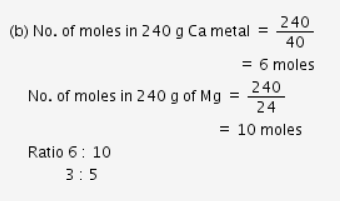# Calculate the molecular mass of CaCO3 (At mass Ca = 40 u, C = 12 u, O = 16 u)

(i) Calculate the molecular mass of CaCO3 (At mass Ca = 40 u, C = 12 u, O = 16 u)
(ii) Verify by calculating that
(a) 5 moles of CO2 and 5 moles of H2O do not have the same mass
(b) 240 g of calcium and 240 g of magnesium elements have a mole ratio of 5:3 (At mass H = 1u, Ca = 40u, Mg = 24u)

(i) Molecular mass of CaCO3 = 40 × 1 + 1 × 12 + 3 × 16
= 40 + 12 + 48
= 100 u.

(ii) (a) CO2 has molar mass = 44 g mol-1
5 moles have molar mass = 44 × 5
= 220 g
H2O has molar mass = 18 g mol-1
= 90 g.